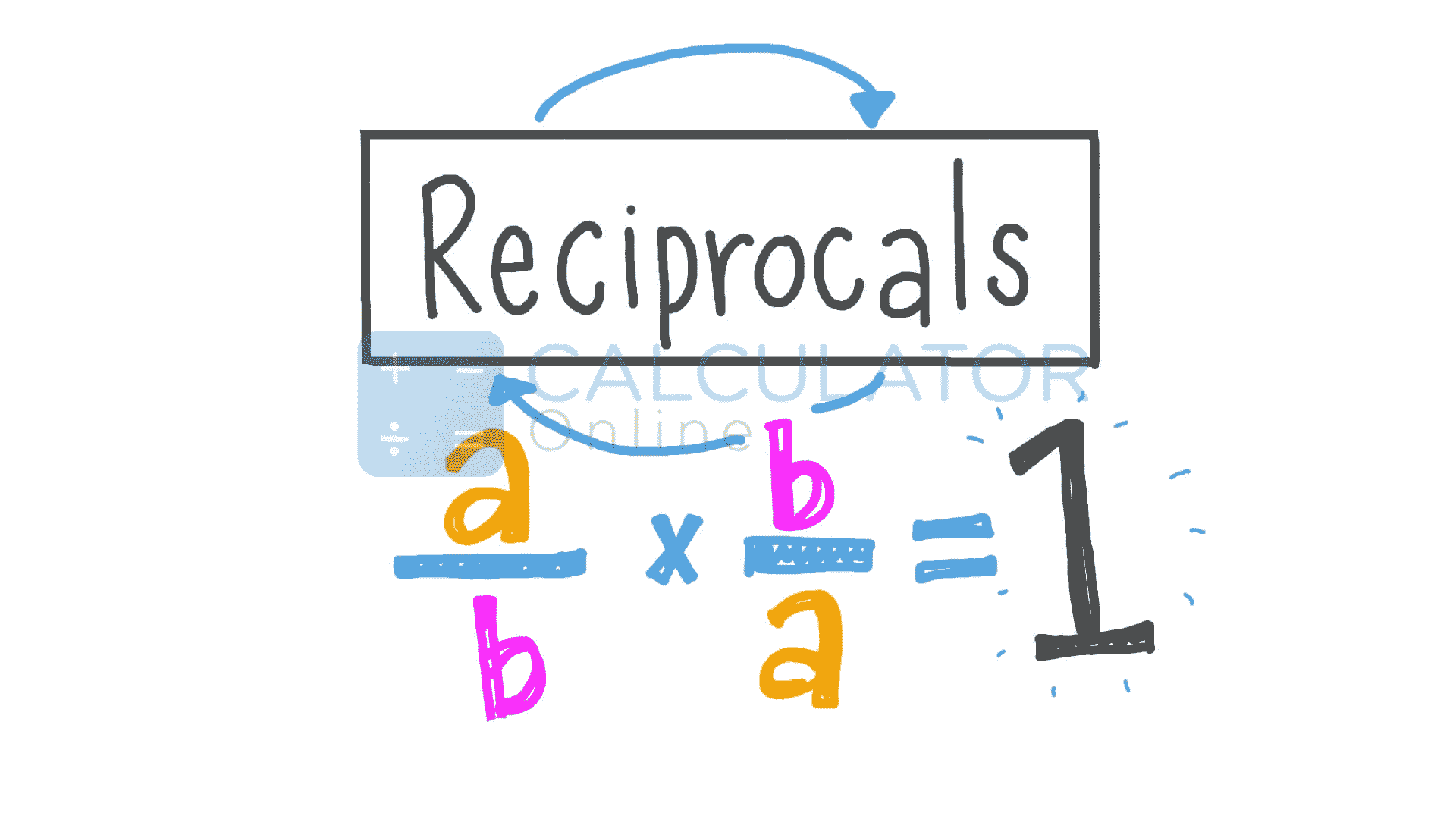•Sign In
• Hire UsUh Oh! It seems you’re using an Ad blocker!

We always struggled to serve you with the best online calculations, thus, there's a humble request to either disable the AD blocker or go with premium plans to use the AD-Free version for calculators.

Or# Reciprocal Calculator

Select input type:

$$\text{Whole Number} \frac{Numerator}{Denominator}$$

$$\frac{Numerator}{Denominator}$$

Whole Number:

Numerator:

Denominator:

Table of Content

 1 What is priceeight Class? 2 priceeight Class Chart: 3 How to Calculate priceeight Density (Step by Step): 4 Factors that Determine priceeight Classification: 5 What is the purpose of priceeight Class? 6 Are mentioned priceeight Classes verified by the officials? 7 Are priceeight Classes of UPS and FedEx same?

Get the Widget!

Add this calculator to your site and lets users to perform easy calculations.

Feedback

How easy was it to use our calculator? Did you face any problem, tell us!

The free online Reciprocal Calculator swiftly finds the reciprocal of different types of number, integers, simple fractions and the mixed fractions.The Reciprocal calculator makes it faster and displays the multiplicative inverse in a matter of seconds.

## How to Find the Reciprocal of Fraction?

To find the reciprocal of fractions, the numerator and the denominator of the fractions are interchanged. Suppose a fraction is “a/b”,then how to find the reciprocal of a fraction, we need to understand “a” is our numerator and “b” is our denominator. We have to completely reverse  the numerator and denominator  “a/b” and convert it into the “b/a”. To find the reciprocal of a proper, improper, or a mixed fraction by the reciprocal calculator we can calculate all the steps are examined in detail. So it can become easy for the user to understand the whole procedure of  how to find the reciprocal of a fraction thoroughly.When we are multiplying the reciprocal of fractions, you are finding an answer of unity as both the fractions are inverse to each other. We need to find which kind of fraction we are entering and we enter the values of the numerator and denominator accordingly into the opposite reciprocal calculator.
The simple procedure of finding the reciprocal of fractions, mixed fraction and an integer/decimal is as follows:

#### The Reciprocal of Fraction:

Turns the Numerator to Denominator of Fraction:
20/24 🔀 24/20=1.2

#### The Reciprocal of  Mixed Fraction:

Solve the a proper fraction and then flip the fraction into its reciprocal by the reciprocal calculator

3(⅘)=19/5 🔀 5/19= 1.2

#### The Reciprocal of Integer/Decimal:

Divide the by 1 to the integer or the decimal, then we can find the inverse by the same method like fractions.

24.75/1 🔀 1/24.75= 100/2475 = 4/99

### Example of Reciprocal of Fraction:

The mixed fraction= 3 (5/7)

Solution:

Turn the fraction into a simple fraction by multiplying the “7” by “3” and adding “5”:

We get

Mixed fraction= 3(5/7)=(21+5)/7

We get the improper fraction=26/7

Then we get by converting the numerator to the denominator and vice versa.

26/7, we get  7/26

By multiplying the both  fractions:

26/7*7/26=1, we get “1”as both the fractions are reciprocal to each other. It is easy to find the reciprocal of the fraction by the reciprocal fraction calculator

### Working of the Reciprocal Calculator:

Finding the reciprocal of a fraction to turn the division into the  multiplication and make our calculations simple.

Input:

• Select the input type
• Enter the required values according to the selected field
• Hit the calculate button to find reciprocal.

Output:

To find the reciprocal is important when it comes to divide the fractions and finding the perpendicular line:

• Step by step calculations are shown.

### What is the reciprocal rule of the division?

The simple rule of the division is “Multiply the dividend by the reciprocal of the divisor, simply you need to invert the divisor and multiply it by the dividend. The opposite reciprocal calculator turns the dividend into the divisor.

### What is the reciprocal of unity or “1”?

The reciprocal of the unity or “1” is one itself, as we can write 1/1 (i.e. 1/1 = 1 and 1 × 1 = 1).

### What is the reciprocal of 4/3?

The reciprocal of 4/3 is ¾ and we know (4/3)(¾)=1

### What is meant by the negative of a reciprocal?

A negative reciprocal is a kind of reciprocal that has a negative sign before the number. The negative reciprocal calculator is especially designed to find the reciprocal of negative numbers and fractions.

Examples: -7/1.-4/3, -5(3/2) etc.

### Conclusion:

We need to simplify the fractions and integers by finding their reciprocal, so the division can be converted into the multiplication. We can use the free online reciprocal calculator to find the reciprocal of a fraction or an integer.

### References:

From the source of Wikipedia: Multiplicative inverse, Examples and counterexamples

From the source of expii.com: Explanation, Turn that Frown Upside Down